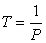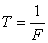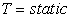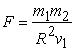﻿ The Relation between Gravity and TimePublications are Open
Access in this journal
Article Versions
Export Article
• Normal Style
• MLA Style
• APA Style
• Chicago Style
Research Article
Open Access Peer-reviewed

### The Relation between Gravity and Time

O.K. AbouEassa
International Journal of Physics. 2019, 7(1), 1-5. DOI: 10.12691/ijp-7-1-1
Received December 05, 2018; Revised January 08, 2019; Accepted January 16, 2019

### Abstract

Newton came upon the idea that there is a universal attractive force of gravity (2). He discovered a fundamental law of gravitation that describes the gravitational attraction between any two bodies (1). In addition, In Einstein’s formulation, gravity is the manifestation of the curvature of spacetime (2). However the relation between gravity and time is poorly recognized. Here I show that any object that rotates on its axis, it generates arms like spiral arms of spiral galaxy and these spiral arms are reflections of gravitational force and shaking in space. The newton equation of gravity and general relativity cannot unify gravity and time. The general theory of relativity can only unify space and gravity. However, if you add velocity or time to Newton’s equation and Einstein’s equation, we can unify gravity with time since velocity is related to time. So, there are three important factors that affect gravity and they are the mass of objects, the speed of rotation of object on its axis and the curvature of spacetime.

### 1. Introduction

1.1. Newtonian Gravity

Some of the earliest investigations in physical science started with questions that people asked about the night sky. Why doesn’t the moon fall to earth? Why do the planets move across the sky? Why doesn’t the earth fly off into space rather than remaining in orbit around the sun? The study of gravitation provides the answers to these and many related questions 1.

It is commonly known that Newton saw an apple fall while he was studying under a tree. In particular, that the apple fell to the earth because it was attracted by earth’s gravity. And Newton went on to reason that exactly in the same manner as the apple falls to the earth, the moon is held in orbit around the earth because of gravitational attraction, and the earth orbits the sun due to the same force of gravity 2. Newton discovered in the 17th century that the same interaction that makes an apple fall out of a tree also keeps the planets in their orbits around the sun 1.

Newton postulated a law of universal gravitation according to which each body in the universe was attracted toward every other body by a force that was stronger the more massive the bodies and the closer they were to each other. Newton went on to show that, according to his law, gravity causes the moon to move in an elliptical orbit around the earth and causes the earth and the planets to follow elliptical paths around the sun. Newton realized that, according to his theory of gravity, the stars should attract each other, so it seemed they could not remain essentially motionless 5.

Every particle of matter in the universe attracts every other particle with a force that is directly proportional to the product of the masses of the particles and inversely proportional to the square of the distance between them 1. Newtonian forces can act instantaneously over arbitrarily large distances. Consider for example, Newton’s gravitational force FG between two masses m1 and m2, separated by a distance R; there is an instantaneous force, as shown in (Figure 1) given by(1)

Where G is Newton’s gravitational constant. (2)

• Figure 1. In classical physics, a force is instantaneous (2)

Newton’s laws of motion, combined with the force of gravitation given by the first equation yield Kepler’s three laws of planetary motion — and was one of the outstanding successes of Newtonian physics. (2)

1.2. General Relativity

In addition, the big puzzle for Einstein was how to modify Newton’s law of gravitation and thus make it consistent with relativity. Einstein’s greatest achievement is that, in 1916, he could go on to propose the general theory of relativity which is consistent with the special theory of relativity and provides an explanation of gravity radically different from Newton’s theory. 2

Until the advent of Einstein’s theory of gravity, space and time were considered to be an empty ‘stage’ given a priori, a precursor to material phenomena. In fact Newton thought of space as an absolute and unchanging structure with an inertial frame being in uniform motion relative to an absolute and immovable space. Newton also considered time to be identical for all observers, flowing at the same rate everywhere in the Universe. With the advent of Einstein all that changed 2.

Einstein, after a long period of intense effort from 1905 to 1915, completed his theory of gravity in 1916 and concluded that matter-energy curves spacetime and matter moves on geodesics that are determined by the curvature of spacetime 2.

Einstein sought the answer to a question that must have seemed to his contemporaries, and all who preceded him, as of no consequence: What meaning is attached to the absolute equality of inertial and gravitational masses? If all bodies move in gravitational fields in precisely the same way, no matter what their constitution or binding forces, then this means that their motion has nothing to do with their nature, but rather with the nature of spacetime. And if spacetime determines the motion of bodies, then according to the notion of action and reaction, this implies that spacetime in turn is shaped by bodies and their motion. 7

The mass of a steel ball is the analog to the matter-energy of a star or a galaxy. There is a quantity, denoted by T and called the energy-momentum tensor, that mathematically represents the total matter-energy-momentum content of a star and must at least contain the energy-momentum four dimensional vector. Furthermore, there are stresses and strains in a medium that contain energy, and these are also included in T. 2

A major breakthrough made by Einstein in understanding the nature of gravity was to connect his intuitive ideas of curved spacetime with the mathematics of curved manifolds discovered by Riemann. Einstein could connect the purely mathematical and formal framework of Riemann geometry with the physical content of spacetime, and which led him to putting down the following fundamental equation relating geometry to matter 2.(2)

Special relativity is a purely kinematical theory without directly involving mass or force. It only deals with uniform motion without the complication of acceleration. The dynamic theory that takes these factors into account is known as general relativity. Whereas special relativity reveals the four dimensional nature of space-time general relativity demands non-Euclidean geometry 9.

In practice, what often happens is that a new theory is devised that is really an extension of the previous theory. For example, very accurate observations of the planet Mercury revealed a small difference between its motion and the predictions of Newton’s theory of gravity. Einstein’s general theory of relativity predicted a slightly different motion from Newton’s theory. The fact that Einstein’s predictions matched what was seen, while Newton’s did not, was one of the crucial confirmations of the new theory. However, we still use Newton’s theory for all practical purposes because the difference between its predictions and those of general relativity is very small in the situations that we normally deal with. Newton’s theory also has the great advantage that it is much simpler to work with than Einstein’s 5.

• Figure 2. The curvature of space due to two masses . It is believed that it is affected by down gravity

### 2. Results and Discussion

2.1. The Relation between Gravity and Time

The newton equation of gravity and general relativity cannot unify Gravity with time. The general theory of relativity can only unify space and gravity. So, it has to be another theory of gravity to generalize the universe as a whole and in a relation with velocity and time.

Rotation is a universal phenomena and many galaxies are rotating. Furthermore, our galaxy has a supermassive black hole at its center. Moreover, Spiral galaxies have copious amounts of gas and dust 2 (Figure 3A). The arrangement of gas and dust in a galaxy represents spiral arms. Any object that rotates on its axis, it generates arms like the spiral arms of a spiral galaxy. It is believed that these spiral arms are reflections of gravitational force. The rotation of the sun on its axis and rotation of the sun around the galactic core are really suggested to be affected by the supermassive black hole rotation in the center of the galaxy 3.

It is believed that spiral arms are also generated by the sun and planets. They represents gravitational force that is caused by any rotated object on its axis (Figure 3B). It is also have another function which is the rotation of the attracted object on its axis. These two functions cannot be obtained if the density of the object below certain value when compared to the other object. When the sun rotates on its axis, the latter two functions of spiral arms are considered. Spiral arms represent the stirring or shaking in space. When the stirring or shaking of an object (like the sun) is fast, the attracted objects which move about it cannot fall into the center and the objects will continue rotating about it (Figure 3) 3.

• Figure 3. A: spiral galaxy and its arms. (Wikipedia), B: Diagram of spiral arms of each body in space represent shaking in space which is a fundamental for gravitational force (3)

Assume there is liquid in a container, and the center of the liquid is representing the sun. When you shake the liquid in the container it produces a hole in the liquid and that represents space-time curvature (Figure 2) and also when you shake well and fast the peripheral liquid cannot fall into the center of the container so the equation need velocity addition. In addition, when you shake fast the liquid goes to the peripheral side of the container, so time will increase and the calculations of days and years will be more and it is also suggested that the speed of the peripheral liquid will be less, so there is an inversely proportional relation between gravity and velocity.

• Figure 4. The gravitational force for all planets except Venus and Uranus
• Figure 5. The gravitational force for Venus & Uranus
2.2. The Definition of Time

Relativity was born out of attempts to relate the descriptions of electrodynamic phenomena in different inertial frames. Einstein’s fundamental paper on special theory of relativity, published in 1905, is titled “Electrodynamics of Moving Bodies” 10. In the flat spacetime of special relativity, observers moving at different speeds will have different measures of time 5 since time shrinks in the state of speed of light.

Einstein merely postulated that the idea of absolute space was not necessary for the new theory. As a result, any questions about absolute uniform motion and absolute rest were regarded as meaningless. Einstein did not answer the fundamental question of why there is no absolute space and therefore no absolute uniform motion and absolute rest, but at least he explicitly stated that special relativity did not need absolute space 11.

To explain time in different manner, the momentum of the earth or any object is related to time because of the more of the momentum of rotation of the earth around the sun, the less time we feel on the earth and time produced on the earth will shrink and vice versa. It is believed that the days and years calculation will be the same or will be less and the feeling of time on the earth will go faster. So, you cannot escape from time because every object that circulating about other object or have momentum in space, it generates time. From this explanation, it is concluded that time is inversely proportional to momentum 3.(3)

Where P is the momentum of an object (space).

When Werner Heisenberg formulated his famous uncertainty principle, he noted that Planck's hypothesis implies that the more accurately one tries to measure the position of a particle, the less accurately one can measure its speed, and vice versa 6. Also, it is implies that the more tightly constrained the value of one observable is, the more uncertain the value of the conjugate variable must be. It follows that the position of a particle that has well-defined momentum is maximally uncertain. In other words, in its ground state the particle is as stationary and as close to the origin as the uncertainty principle permits; there is a conflict between the advantage energetically of being near the origin, and the energetic penalty that the uncertainty principle exacts for having a well-defined position 8.

This means that we cannot exactly predict the position of a particle in a certain time since the momentum is related to the time from the following equation. In addition, we cannot precisely predict the position of the electron in the future because we cannot determine all the causes that affects the changing of the position of a particle in a certain time and if we could determine them, we cannot predict what will happen in a large ratio due to the complexity of the issue when the effectors or the causes are several 3.

There is a chance that the changing of a position of a particle will be nearer or farther and there will be a new position after that and that is why it is not accurate if there is a relation between two particles. Also, it is suggested you need to add the direction of one particle to another. It is believed that the equation (4) can unify the uncertainty principle with special relativity and the new theory of time. The equation (4) can be generalized on everything in the universe including the atoms.(4)

In addition, if it is suggested that time is inversely proportional to momentum 3, so time is direct proportional to static, so time is the static state. It is believed that there is no one is immortal other than the divine creator which proves his existence, so time is the divine creator and the divine creator is time. It is concluded that there is a divine creator who is immortal and has power to all times, and rotating about him everything in the universe.

It is believed the existence, immortality and divinity of the creator. The divine creator has power on everything including time, gravity and the entire universe. There is only "one" in the universe and that “one” must be the divine creator.(5)
• Figure 6. The relation between static and time
2.3. A More Unification Equation between Gravity and Time

The rate of flow of time is fastest in empty space, and slows down in the presence of matter-energy 2. So, gravity is proportional to time and gravity is inversely proportional to velocity.

Moreover, Einstein’s theory of gravity cannot explain how space and time come into existence 2, so we need velocity or time addition to the equation to relate gravity with velocity and time since velocity is related to time from equation (3).(6)

Where v is the speed of rotating of an object on its axis.

To unify gravity with time is that the more the gravity of the sun the more the distance between the sun and the earth so the calculations of the days of the year will be more, so time will increase. Also, it is suggested that gravity creates time to any system.

In addition, there are three factors that affect gravity; the mass of objects, the speed of rotation of the sun on its axis and the curvature of space-time. The last factor is a result of rotated objects on its axis.

From equation (1), (2), (6) it is concluded a more unifying equation(7)

It is suggested that the heart of human being is near to the left and not in the center of the body because when the earth is rotating on its axis it pumps the blood to right more than to the left, so it needed to be slightly to the left to increase pumping the blood to that side.

### 3. Conclusion

It is concluded that we can unify gravity with time into one equation if you add the velocity or time, and we can unify Newton’s theory of gravity with special relativity, general relativity and time. Also, it is concluded that any rotated object on its axis generates the spiral arms like a spiral arms of a galaxy and that generates gravity and time to any system.

### References

  Pearson Education Inc., 2016. Hugh D. Young & Roger A. Freedman. Sears & Zemansky's University Physics with Modern Physics, 14th edition. In article  World Scientific Publishing Co., 2015. Belal E Baaquie & Frederick H Willeboordse. Exploring the Invisible Universe from Black Holes to Superstrings. In article  Osama Khalil. "Theoretical Atomic Model and the Theory of Everything". International Journal of Physics, Vol. 5, No. 3, 87-91. 2017. In article  Osama Khalil. “Time Concept and the Center of the Universe”. International Journal of Physics, vol. 5, No. 5, 154-156. 2017. In article  Bantam Book, 1987. Stephen Hawking. A Brief History of Time. In article  Bantam Press, 2001. Stephen Hawking. The Universe in a Nutshell. In article  Springer, 2007. Norman K. Glendenning. Special and General Relativity with Applications to White Dwarfs, Neutron Stars and Black Holes, First Edition. In article  Oxford University Press, 2014. James Binney & David Skinner. The Physics of Quantum Mechanics. In article  Springer, 2010. Jan C.A. Boeyens. Chemical Cosmology. In article  Hindustan Book Agency, 2009. Pankaj Sharan. Spacetime, Geometry and Gravitation. In article  Springer-Verlag Berlin Heidelberg, 2009. Vesselin Petkov. Relativity and the Nature of Spacetime, Second Edition. In articleThis work is licensed under a Creative Commons Attribution 4.0 International License. To view a copy of this license, visit http://creativecommons.org/licenses/by/4.0/